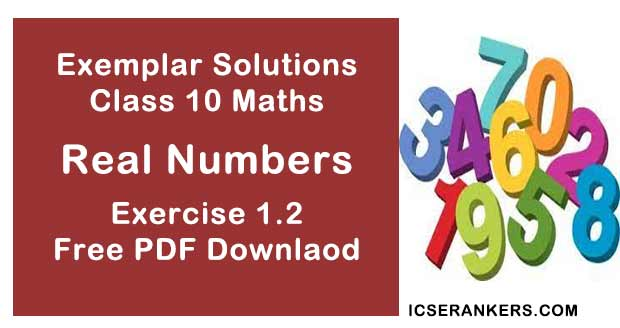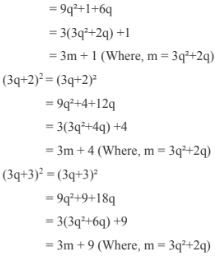# Chapter 1 Real Number NCERT Exemplar Solutions Exercise 1.2 Class 10 MathsChapter Name NCERT Maths Exemplar Solutions for Chapter 1 Real Numbers Exercise 1.2 Book Name NCERT Exemplar for Class 10 Maths Other Exercises Exercise 1.1Exercise 1.3Exercise 1.4 Related Study NCERT Solutions for Class 10 Maths

### Exercise 1.2 Solutions

1. Write whether every positive integer can be of the from (4q + 2), where q is an integer. Justify your answer.

Solution

‘No’
We know that,
Dividend = divisor × quotient + remainder
According to Euclid’s division lemma,
a = bq + r
If, b = 4 then,
a = 4q + r
So,
r = 0, 1, 2, 3 (as r is positive and less than 4)
We get,
4q, 4q + 1, 4q + 2 and 4q + 3 respectively.
So, every positive integer cannot be only in the form of 4q+2.

2. “The product of two consecutive positive integers is divisible by 2”. Is this statement true or false? Give reasons.

Solution

This statement is true.
For any two consecutive numbers one will be even and the other will be odd. Let n, (n+ 1).
So, their product is always multiple of 2.
Hence, the product of two consecutive positive integers is divisible by 2.

3. “The product of three consecutive positive integers is divisible by 6”. Is this statement true or false? Justify your answer.

Solution

The statement is true.
Three consecutive positive integers are n, (n + 1), (n + 2).
In those 3 consecutive integers, one will be even and other will be divisible by 3.
Therefore, the product of three will be divisible by 6,
For e.g. 16, 17, 18
Here 16 is even, and18 is divisible by 3.
So, 16 × 17 × 18 is divisible by 6.

4. Write whether the square of any positive integer can be of the form of (3m + 2), where m is a natural number. Justify your answer.

Solution

No, the square of any positive integer cannot be written in the form 3m + 2 where m is a natural number
Explanation:
By Euclid’s division lemma, we have
a = bq + r, where b, q and r are positive integers,
For b = 3
a = 3(q) + r,
For r = 0, 1, 2, 3
So, 3q + 0, 3q + 1, 3q + 2, 3q + 3 are positive integers,
According to question, the square of any positive integer can be written as,
(3q)2 = 9q2
= 3(3q)2 = 3m (where 9q2 = m)
(3q + 1)2 = (3q + 1)25. A positive integer is of the form (3q + 1), q being a natural number. Can you write its square in any form other than (3m + 1) i.e., 3m or (3m + 2) for some integer m? Justify your answer.

Solution

No.
(3q + 1)2 cannot be expressed in any other form other than 3m + 1.
Explanation :
Consider the positive integer 3q + 1, so,
(3q + 1)2 = 9q2 + 6q +  1
= 3 (3q2 +2q) + 1
= 3m + 1, (where m is an integer = 3q2 + 2q)
Hence, (3q  + 1)2 cannot be expressed in any other form other than 3m + 1.

6. The numbers 525 and 3000 are both divisible only by 3, 5, 15, 25 and 75, what is HCF of (3000, 525)? Justify your answer.

Solution

The numbers 525 and 3000 both are divisible only by 3, 5, 15, 25 and 75. So, highest common factors out of 3, 5, 15,  25 and 75 is 75.
So, HCF of (525, 3000) is 75.
Justification :
525 = 5 ×5 × 3 × 7
= 3× 52  × 71
3000 = 23 × 53 ×31
= 23 × 31 × 53
Therefore,
HCF = 31 × 52 = 75
Hence, justified.

7. Explain why 3 × 5 × 7 + 7 is a composite number.

Solution

A number which is not prime is composite.
3 × 5 × 7 + 7 = 7[3 × 5 + 1]
= 7[15 + 1]
= 7 × 16
So, it have factors = 7 × 2 × 2 × 2 × 2
As the factors are other than one and itself, so, the number is not prime.
Hence, the number (3 × 5 × 7 + 7) is composite.

8. Can two numbers have 18 as their HCF and 380 as their LCM? Give reasons.

Solution

380 and 18 are not the LCM and HCF of any two numbers.
We know that,
HCF (x, y) × LCM (x, y) = (x × y)
So, 18 must be factor of 380.
But, 380 is not divisible by 18.
Hence, 380 and 18 are not the LCM and HCF of any two numbers.

9. Without actually performing the long division, find if 987/10500 will have terminating or non-terminating (repeating) decimal expansion. Give reasons for your answer.

Solution

The denominator has prime factors only in 2 and 5 so, the number is terminating decimal. On solving we get,  987/10500  = 0.094

10. A rational number in its decimal expansion is 327.7081. What can you say about the prime factors of q, when this number is expressed in the form p/q? Give reasons.

Solution

327.7081 is terminating decimal in the form of
p/q = 3277081/10000
q = 24 × 54
As, q has only multiples of 2 and 5 so it is terminating decimal.